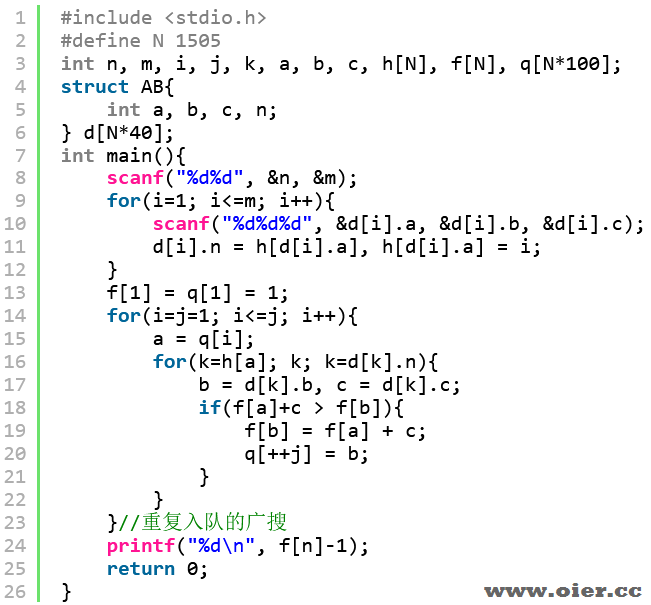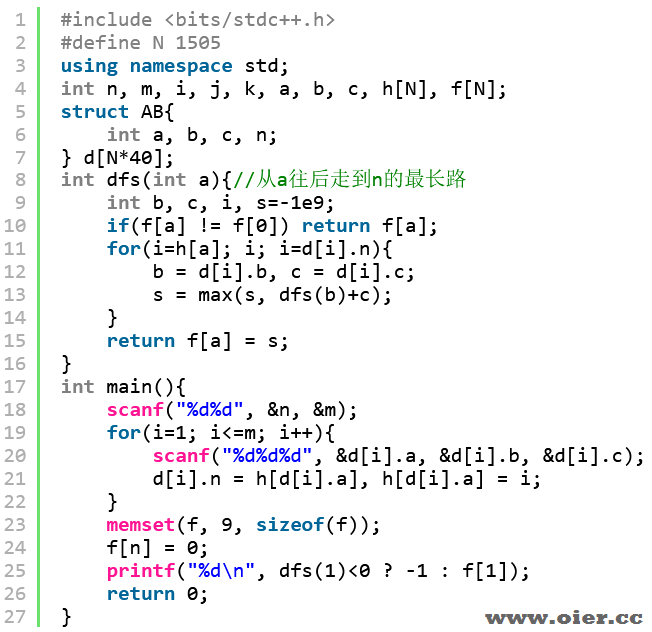324+

## 输入输出样例

### 输入样例 #1

2 1
1 2 1

### 输出样例 #1

1

## 说明

– 对于 $20\%$的数据，$n \leq 100$，$m \leq 10^3$。
– 对于 $40\%$ 的数据，$n \leq 10^3$，$m \leq 10^{4}$。
– 对于 $100\%$ 的数据，$1 \leq n \leq 1500$，$1 \leq m \leq 5 \times 10^4$，$1 \leq u, v \leq n$，$-10^5 \leq w \leq 10^5$。

## 程序实现